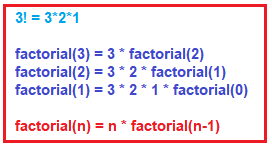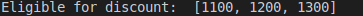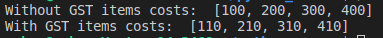# Recursive and Lambda Functions in Python

## Recursive and Lambda Functions in Python with Examples

In this article, I am going to discuss Recursive and Lambda Function in Python with Examples. Please read our previous article where we discussed Types of Variables in Python with examples. As part of this article, you will understand the following pointers which are related to Lambda and Recursive Function in Python.

1. Recursive Function in Python
2. Lambda Function (Anonymous Functions) in Python
3. filter() function in Python
4. map() function in Python
5. reduce() function in Python
##### Recursive Function in Python

A function is called recursive when it is called by itself. Let’s understand this with an example. Let’s consider a function which calculates the factorial of a number. It can be written as a recursive functions as explained below.###### Example: Factorial using the recursive function in python (Demo33.py)
```def factorial(n):
if n ==0:
result=1
else:
result = n * factorial(n-1)
return result

x= factorial(4)
print("Factorial of 4 is: ",x)```

Output: Factorial of 4 is: 24

###### Example: Factorial without recursive function in python (Demo34.py)
```def f1():
n=4
fact=1
while(n>0):
fact=fact*n
n=n-1
print("Factorial of the number is: ", fact)

f1()```

Output: Factorial of the number is: 24

From the above two programs we can conclude that using recursive functions brings in some advantages:

1. We can reduce the length of the code and improves readability
2. We can solve complex problems in a very easy way.
##### Lambda Function (Anonymous Functions) in Python

The name of the function is the mandatory item in the function definition as discussed earlier. But, in python, we have a keyword called ‘lambda’ with which we can define a simple function in a single line without actually naming it. Such functions are called lambda functions.

Syntax: lambda argument_list: expression

###### Example: Lambda Function in Python (Demo35.py)
```s = lambda a: a*a
x=s(4)
print(x)```

Output: 16

###### Example: Lambda Function in Python (Demo36.py)
```add = lambda a,b: a+b
print(x)```

Output: 9

##### Points to Remember while working with Python Lambda Functions:
1. A lambda function can take any number of arguments but should have only one expression.
2. It takes the parameters and does some operation on them and returns the result, just the same as normal functions.
3. The biggest advantage of lambda functions is that a very concise code can be written which improves the readability of the code.
4. This can be used for only simple functions but not for complex functions.
5. The lambda function, mostly, can be used in combination with other functions such as map(), reduce(), filter() etc which we are going to discuss now.
##### filter() function in Python:

This function is used to filter values from a sequence of values. The syntax is:

Syntax: filter(function, sequence)

The filter function will filter the elements in the sequence based on the condition in the function. Let’s understand it through the example.

###### Example: Filter Function in python (Demo37.py)
```items_cost = [999, 888, 1100, 1200, 1300, 777]
gt_thousand = filter(lambda x : x>1000, items_cost)
x=list(gt_thousand)
print("Eligible for discount: ",x)```

Output:In the above example, the filter applies the lambda function on all the elements in the ‘items_cost’ and returns the elements which satisfy the lambda function.

##### map() function in Python:

This function is used to map a particular function onto the sequence of elements. After applying, this returns a new set of values.

Syntax: map(function, sequence)

###### Example: Map Function in Python (Demo38.py)
```without_gst_cost = [100, 200, 300, 400]
with_gst_cost = map(lambda x: x+10, without_gst_cost)
x=list(with_gst_cost)
print("Without GST items costs: ",without_gst_cost)
print("With GST items costs: ",x)```
###### Output:##### reduce() function in Python:

This function reduces the sequence of elements into a single element by applying a specific condition or logic. To use the reduce function we need to import the functools module.

Syntax: reduce(function, sequence)

###### Example: Reduce Function (Demo39.py)
```from functools import reduce
each_items_costs = [111, 222, 333, 444]
total_cost = reduce(lambda x, y: x+y, each_items_costs)
print(total_cost)```

Output: 1110